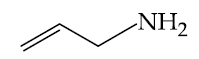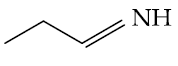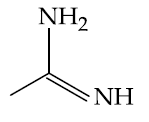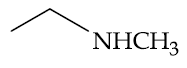## Filters

Sort by :
Clear All
Q
Engineering
357 Views   |

The increasing order of nitration of the following compounds is :• Option 1)

(b) < (a) <  (c) < (d)

• Option 2)

(a) < (b) <  (c) < (d)

• Option 3)

(b) < (a) < (d) < (c)

• Option 4)

(a) < (b) < (d) < (c)

As we learnt in  Gabriel Phthalimide Synthesis - Further alkylation can be stopped and a pure primary amine can be obtained by alkylation of phthalimide ( Gabriel synthesis ) followed by hydrolysis. - wherein     Here the aniline is least reactive due to formation of anilinium ion in acidic medium.    Option 1) (b) < (a) <  (c) < (d) Option 2) (a) < (b) <  (c) < (d) Option 3) (b) < (a) <...
Engineering
100 Views   |

The increasing order of basicity of the following compounds is:

(a)(b)(c)(d)• Option 1)

(d) < (b) < (a) < (c)

• Option 2)

(a) < (b) < (c) < (d)

• Option 3)

(b) < (a) < (c) < (d)

• Option 4)

(b) < (a) < (d) < (c)

As we learned

Comparision of Basic nature in aliphatic amines in gaseous phase -

The +I effect of the alkyl group, which increase the electron density on N atom, facilitates the nitrogen atom to use its unshared pair of electrons for bond formation. Thus R - NH2 is expected to be more basic than NH3. Going by the same arguments the basic strength of amines is expected to increase with the increase in the number of alkyl groups attached to the nitrogen atom.

- wherein

(b) –lone pair present on  hybridized nitrogen

(a)  of  hybridized carbon

(d)  of two alkyl group

(c) Guanidine derivative

Option 1)

(d) < (b) < (a) < (c)

Option 2)

(a) < (b) < (c) < (d)

Option 3)

(b) < (a) < (c) < (d)

Option 4)

(b) < (a) < (d) < (c)

View More
Engineering
414 Views   |

The increasing order of diazotisation of the
following compounds is :• Option 1)

(a) < (b) < (c) < (d)

• Option 2)

(a) < (d) < (b) < (c)

• Option 3)

(a) < (d) < (c) < (b)

• Option 4)

(d) < (c) < (b) < (a)

As we have learnt,   Fate of aliphatic diazonium ion - The diazonium ions of aliphatic amines are very unstable and produces carbocation immediately, which can produce different products.  - wherein     Fate of aromatic diazonium ion - Diazonium salts of aromatic amines are comparatively more stable and evolve nitrogen only on heating.These diazonium salts can be isolated at low...
Engineering
92 Views   |

Products A and B formed in the following reactions are respectively :• Option 1)• Option 2)• Option 3)• Option 4)As we have learned

Reaction with Nitrous Acid Primary Amines -

Primary amines react with nitrous acid to produce diazonium ion.

- whereinOption 1)This is incorrect

Option 2)This is incorrect

Option 3)This is incorrect

Option 4)This is correct

View More
Exams
Articles
Questions